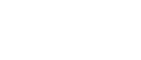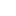#• PRODUCTS• SOLUTIONS• TUTORIALS• SUPPORTGlobal | English• Global
• United States
• Europe
• China
• Korea
• Japan

#• PRODUCTS
• SOLUTIONS
• TUTORIALS
• SUPPORT

# Linear Hall Effect ICs

Magnetic Sensors

Linear Hall Effect ICs are composed of Hall element and an amplifier in one package.
They output the analog voltage proportional to the magnetic field (magnetic flux density.)

## Linear Hall ICs

These products have the fixed magnetic sensitivity (gain) product by product.

## Programmable Linear Hall ICs

These products have EEPROM inside. Customers can adjust magnetic sensitivity and offset voltage freely.

## Lineup

Linear Hall Effect ICs

`Part Number`
```Part
Status```
```Power Supply
Voltage (MIN.)
[V]```
```Power Supply
Voltage (TYP.)
[V]```
```Power Supply
Voltage (MAX.)
[V]```
```Sensitivity (MIN.)
[mV/MT]```
```Sensitivity (TYP.)
[mV/MT]```
```Sensitivity (MAX.)
[mV/MT]```
`Output`
```Response time
[μsec]```
`Other`
```Operating
Temperature
[℃]```
```Device
Package```
```Number
of Pins```
`EG230L`
`EOL`
`4.75`

`5.25`
`5`

`40`
`Analog`
`2`
`Automotive grade`
`-40 to 125`
`SIP`
`3`
`EQ0321`
`EOL`
`2.7`

`5.5`

`Analog`

`-30 to 85`
`SON`
`10`
`EQ430L`
`MP`
`3`
`5`
`5.5`
`110`
`130`
`150`
`Analog`
`4`

`-40 to 100`
`SOT`
`3`
`EQ431L`
`MP`
`3`
`5`
`5.5`
`55`
`65`
`75`
`Analog`
`3`

`-40 to 100`
`SOT`
`3`
`EQ432L`
`MP`
`3`
`5`
`5.5`
`34`
`40`
`46`
`Analog`
`3`

`-40 to 100`
`SOT`
`3`
`EQ433L`
`MP`
`3`
`5`
`5.5`
`17`
`20`
`23`
`Analog`
`2`

`-40 to 100`
`SOT`
`3`
`EQ730L`
`MP`
`3`
`5`
`5.5`
`110`
`130`
`150`
`Analog`
`2`

`-40 to 100`
`SIP`
`3`
`EQ731L`
`MP`
`3`
`5`
`5.5`
`55`
`65`
`75`
`Analog`
`1`

`-40 to 100`
`SIP`
`3`
`EQ732L`
`MP`
`3`
`5`
`5.5`
`34`
`40`
`46`
`Analog`
`1`

`-40 to 100`
`SIP`
`3`
`EQ733L`
`MP`
`3`
`5`
`5.5`
`17`
`20`
`23`
`Analog`
`1`

`-40 to 100`
`SIP`
`3`
`EQ950L`
`EOL`
`4.5`

`5.5`
`15`

`130`
`Analog`
`1`

`-40 to 105`
`SIP`
`5`